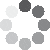Enter parameters Rectangle
Enter parameters
Length:
ft
• inches (in)
• feet (ft)
• yards (yd)
• centimeters (cm)
• meters (m)
Depth 1:
ft
• inches (in)
• feet (ft)
• yards (yd)
• centimeters (cm)
• meters (m)
Width:
ft
• inches (in)
• feet (ft)
• yards (yd)
• centimeters (cm)
• meters (m)
Depth 2:
ft
• inches (in)
• feet (ft)
• yards (yd)
• centimeters (cm)
• meters (m)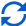Result
Volume:
0
US gal
• US Gallons (US gal)
• UK Gallons (UK gal)
• Liters (l)
Surface area:
0
ft²
• square feet (ft²)
• square inches (in²)
• square yards (yd²)
• square miles (mi²)
• acres (ac)
• square centimeters (cm²)
• square meters ()
Enter parameters
Base:
ft
• inches (in)
• feet (ft)
• yards (yd)
• centimeters (cm)
• meters (m)
Depth:
ft
• inches (in)
• feet (ft)
• yards (yd)
• centimeters (cm)
• meters (m)
Height:
ft
• inches (in)
• feet (ft)
• yards (yd)
• centimeters (cm)
• meters (m)Result
Volume:
0
US gal
• US Gallons (US gal)
• UK Gallons (UK gal)
• Liters (l)
Surface area:
0
ft²
• square feet (ft²)
• square inches (in²)
• square yards (yd²)
• square miles (mi²)
• acres (ac)
• square centimeters (cm²)
• square meters ()
Enter parameters
Diameter:
ft
• inches (in)
• feet (ft)
• yards (yd)
• centimeters (cm)
• meters (m)
Depth:
ft
• inches (in)
• feet (ft)
• yards (yd)
• centimeters (cm)
• meters (m)Result
Volume:
0
US gal
• US Gallons (US gal)
• UK Gallons (UK gal)
• Liters (l)
Surface area:
0
ft²
• square feet (ft²)
• square inches (in²)
• square yards (yd²)
• square miles (mi²)
• acres (ac)
• square centimeters (cm²)
• square meters ()
Enter parameters
Length:
ft
• inches (in)
• feet (ft)
• yards (yd)
• centimeters (cm)
• meters (m)
Depth:
ft
• inches (in)
• feet (ft)
• yards (yd)
• centimeters (cm)
• meters (m)
Width:
ft
• inches (in)
• feet (ft)
• yards (yd)
• centimeters (cm)
• meters (m)Result
Volume:
0
US gal
• US Gallons (US gal)
• UK Gallons (UK gal)
• Liters (l)
Surface area:
0
ft²
• square feet (ft²)
• square inches (in²)
• square yards (yd²)
• square miles (mi²)
• acres (ac)
• square centimeters (cm²)
• square meters ()(1 votes, average: 5.00 out of 5)Loading...
Similar Calculators:
Real-time graphics. Make the calculations and see the changes.
Real-time graphics. Make the calculations and see the changes.
Real-time graphics. Make the calculations and see the changes.
Real-time graphics. Make the calculations and see the changes.
Embed
Similar Calculators:If you are reading this article, then it is likely that you are trying to calculate how much water your swimming pool contains.

We understand that estimating this by eye is almost impossible!

Don’t worry – our online pool volume calculator can help! 😇

Read on to see how easy it is to use and how it can help you efficiently calculate the total capacity of your pool.

In addition, we will go through the calculations.

Contents:

## Pool volume calculator formula

Calculate the total capacity of your swimming pool by entering your dimensions in metric units (centimeters or meters) or imperial units (yards, feet or inches).

The pool size calculator will then estimate the surface area, volume and liquid capacity using the below formulas:

### Rectangular Pool with Constant Depth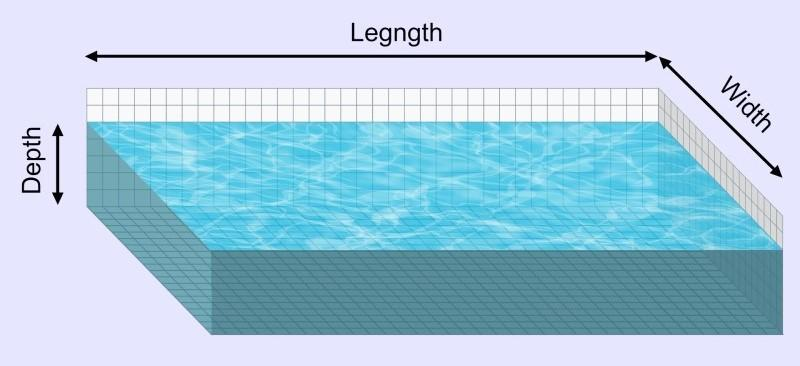For a rectangular swimming pool, the surface area is calculated using the formula:

$$Surface\,Area = Length × Width$$

and volume is calculated by:

$$Volume = Surface\,Area × Depth$$

### Rectangular Pool with Variable Depth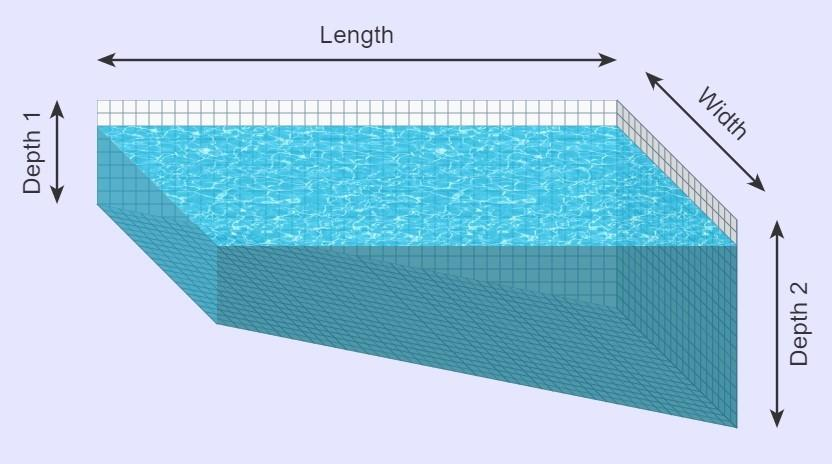For a rectangular swimming pool with variable depth the surface area is calculated using the formula:

$$Surface\,Area = Length × Width$$

and volume is calculated by:

$$Volume = Surface\,Area × Average\,Depth$$

where

$$Average\,Depth = {Depth\,1 + Depth\,2 \over 2}$$

### Triangular Pool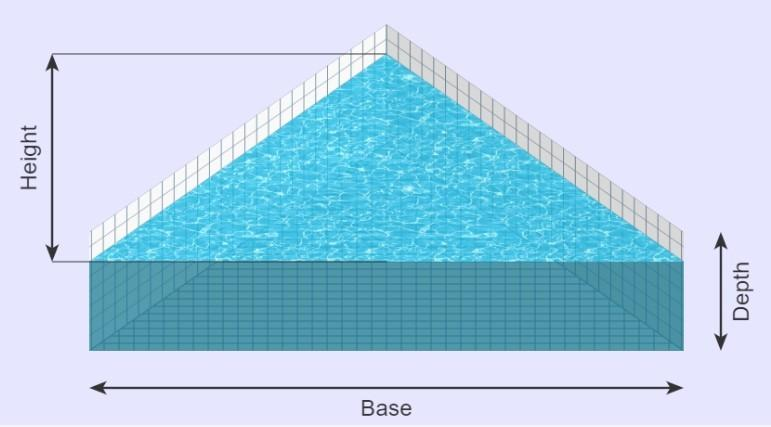For a triangular swimming pool, the surface area is calculated using the formula:

$$Surface\,Area = {Height × Base \over 2}$$

and volume is calculated by:

$$Volume = Surface\,Area × Depth$$

### Circular Pool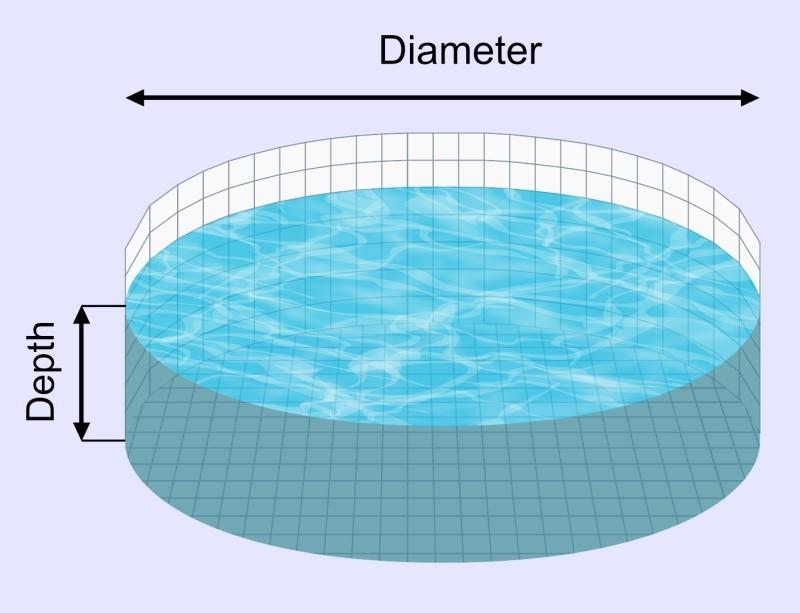For a circular pool, the surface area is calculated using the formula:

$$Surface\,Area= \pi × Radius^2$$

where

$$Radius = {Width \over 2}$$

and volume is calculated by:

$$Volume = Surface\,Area × Depth$$

### Oval Pool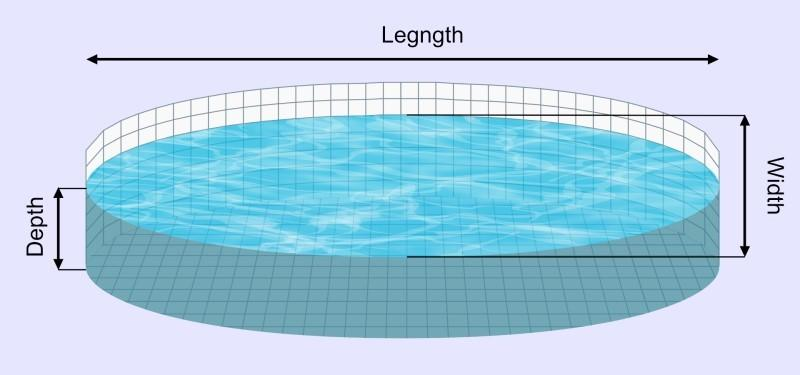For an oval pool, the surface area is calculated using the formula:

$$Surface\,Area= \pi × {Width \over 2} × {Length \over 2}$$

and volume is calculated by:

$$Volume = Surface\,Area × Depth$$

The above calculations will give units in cubic feet.

The calculator then converts this into either US gallons, UK gallons or litres by doing the following conversions:

$$1\,ft^3 = 7.48\,US\,gallons$$

$$1\,ft^3 = 6.23\,UK\,gallons$$

$$1\,ft^3 = 28.32\,litres$$

Note that these are approximations. The calculator does the exact conversion for you. 🙂

Something not clear?

Keep reading for five full examples where we determine the surface area and volume of swimming pools of various shapes and sizes.

Otherwise, simply enter your measurements into our pool size calculator!

## Example Calculations

• Rectangular pool with constant depth

Imagine my pool measures 10 feet in length, 20 feet in width and has a constant depth of 6 feet.

I would enter these measurements into the pool calculator to work out the surface area and total volume of the swimming pool:

$$Surface\,Area = Length × Width = 10\,ft × 20\,ft = 200\,ft^2$$

$$Volume = Surface\,Area × Depth = 200\,ft^2 × 6\,ft = 1200\,ft^3$$

Let’s say that I want to figure out the volume in US gallons.

Using the conversion 1 ft³ = 7.48 US gallons gives:

$$1200\,ft^3 = 8976.62\,US\,gallons$$

• Rectangular pool with varying depth

Let’s now imagine that I have a rectangular above ground swimming pool 20 feet in length and 8 feet in width.

The ground is sloping from 4 feet in the shallow end to 10 feet in the deep end.

I want to estimate how many litres of water will be needed to fill the pool up completely.

I would enter the values in the calculator, which would do the following calculations:

$$Surface\,Area = Length × Width = 20\,ft × 8\,ft = 160\,ft^2$$

$$Average\,Depth = {Depth\,1 + Depth\,2 \over 2} = {4\,ft + 10\,ft \over 2} = 7\,ft$$

Therefore:

$$Volume = Surface\,Area × Average\,Depth = 160\,ft^2 × 7\,ft = 1120\,ft^3$$

and because 1 ft³ = 28.32 litres:

$$1120\,ft^3 = 31714.87\,litres$$

• Triangular pool

Imagine I have a triangular swimming pool measuring 30 feet in height and 10 feet in width.

The base of the pool is at a depth of 5 feet. I wish to calculate how many UK gallons of water are required to completely fill the pool.

Entering these measurements into the calculator gives:

$$Surface\,Area = {Height × Base \over 2} = {30\,ft × 10\,ft \over 2} = 150\,ft^2$$

$$Volume = Surface\,Area × Depth = 150\,ft^2 × 5\,ft = 750\,ft^3$$

Finally, because 1 ft³ = 6.23 UK gallons we have:

$$750\,ft^3 = 4671.63\,UK\,gallons$$

• Circular pool

Imagine I have a circular swimming pool with a width of 50 feet with a constant depth of 8 feet.

To calculate the surface area and total volume of the pool we have:

$$Radius = {Width \over 2} = {50\,ft \over 2} = 25\,ft$$

$$Surface\,Area= \pi × Radius^2 = \pi × (25\,ft)^2 = 1964.495\,ft^2$$

and

$$Volume = Surface\,Area × Depth = 1964.495\,ft^2 × 8\,ft = 15707.96\,ft^3$$

Let’s imagine I want to calculate the swimming pool’s volume in litres.

Recall that one cubic foot is equivalent to  litres.

We can therefore calculate the capacity in litres by multiplying by 28.32:

$$15707.96\,ft^3 = 444800\,litres$$

• Oval swimming pool

For our final example, let’s say that I want to know how many US gallons of water it will take to fill an oval tank that measures 30 feet in width, 20 feet in length and 10 feet deep.

The pool gallon calculator first calculates the surface area:

$$Surface\,Area= \pi × {Width \over 2} × {Length \over 2} = \pi × {30\,ft \over 2} × {20\,ft \over 2} = 471.239\,ft^2$$

and then the volume:

$$Volume = Surface\,Area × Depth = 471.239\,ft^2 × 10\,ft = 4712.39\,ft^3$$

Finally, to convert this into US gallons, recall that one cubic foot of water is equivalent to 7.48 US gallons. We can therefore calculate the capacity in US gallons by multiplying the volume by 7.48:

$$4712.39\,ft^3 = 35248.7\,US\,gallons$$

Note: It is crucial that you don’t round your answers too early! If you round the calculated values for surface area and then volume to the nearest whole numbers, you will calculate an answer that is slightly too big or small. Using our pool calculator will help you avoid doing this!

Finally,

## What if my dimensions aren’t in feet?

Well, the calculator does the conversions for you!

Simply select the desired units in the drop down option and the pool calculator will do the following conversions for you:

$$1\,foot = 12\,inches = 0.33\,yards = 30.48\,centimeters = 0.3048\,meters$$

$$1\,ft^2 = 144\,in^2 = 0.111\,yd^2 = 929\,cm^2= 0.0929\,m^2$$

It’s that simple! 😉

Embed this calculator on your site!Add live graphics
Copied to clipboard! Preview